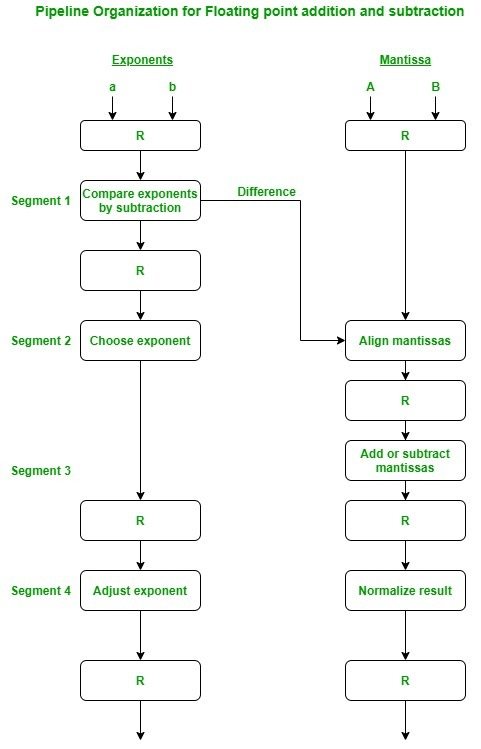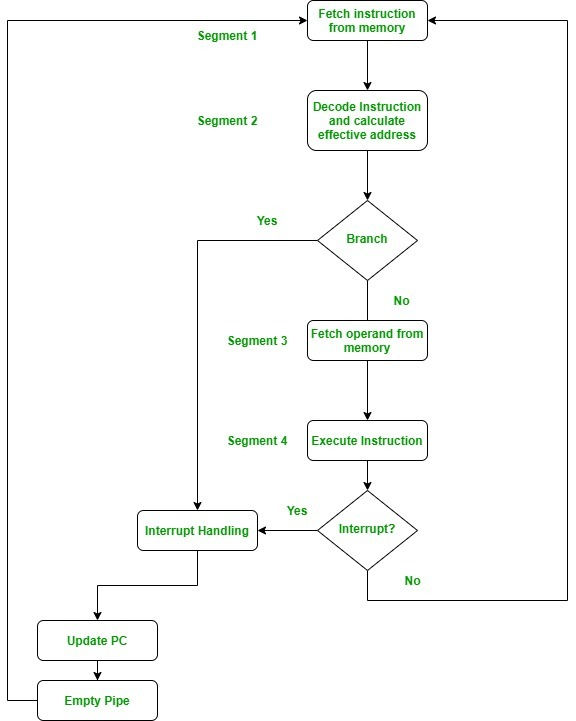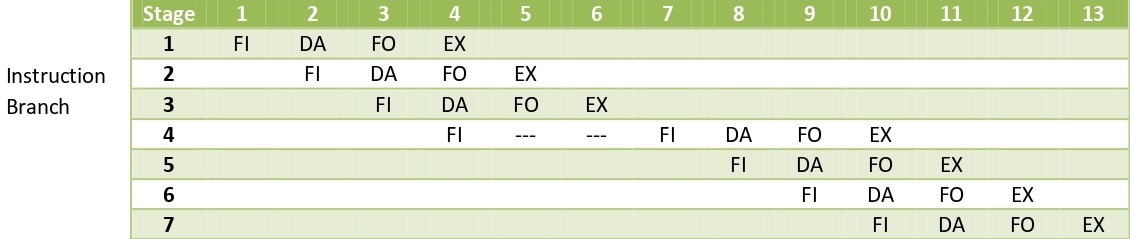Skip to content
Related Articles
Arithmetic Pipeline and Instruction Pipeline
• Difficulty Level : Hard
• Last Updated : 16 May, 2020

1. Arithmetic Pipeline :
An arithmetic pipeline divides an arithmetic problem into various sub problems for execution in various pipeline segments. It is used for floating point operations, multiplication and various other computations. The process or flowchart arithmetic pipeline for floating point addition is shown in the diagram.Floating point addition using arithmetic pipeline :
The following sub operations are performed in this case:

1. Compare the exponents.
2. Align the mantissas.
3. Add or subtract the mantissas.
4. Normalise the result

First of all the two exponents are compared and the larger of two exponents is chosen as the result exponent. The difference in the exponents then decides how many times we must shift the smaller exponent to the right. Then after shifting of exponent, both the mantissas get aligned. Finally the addition of both numbers take place followed by normalisation of the result in the last segment.

Example:
Let us consider two numbers,

`X=0.3214*10^3 and Y=0.4500*10^2 `

Explanation:
First of all the two exponents are subtracted to give 3-2=1. Thus 3 becomes the exponent of result and the smaller exponent is shifted 1 times to the right to give

`Y=0.0450*10^3 `

Finally the two numbers are added to produce

`Z=0.3664*10^3 `

As the result is already normalized the result remains the same.

2. Instruction Pipeline :
In this a stream of instructions can be executed by overlapping fetch, decode and execute phases of an instruction cycle. This type of technique is used to increase the throughput of the computer system. An instruction pipeline reads instruction from the memory while previous instructions are being executed in other segments of the pipeline. Thus we can execute multiple instructions simultaneously. The pipeline will be more efficient if the instruction cycle is divided into segments of equal duration.

In the most general case computer needs to process each instruction in following sequence of steps:

1. Fetch the instruction from memory (FI)
2. Decode the instruction (DA)
3. Calculate the effective address
4. Fetch the operands from memory (FO)
5. Execute the instruction (EX)
6. Store the result in the proper place

The flowchart for instruction pipeline is shown below.Let us see an example of instruction pipeline.

Example:Here the instruction is fetched on first clock cycle in segment 1.
Now it is decoded in next clock cycle, then operands are fetched and finally the instruction is executed. We can see that here the fetch and decode phase overlap due to pipelining. By the time the first instruction is being decoded, next instruction is fetched by the pipeline.

In case of third instruction we see that it is a branched instruction. Here when it is being decoded 4th instruction is fetched simultaneously. But as it is a branched instruction it may point to some other instruction when it is decoded. Thus fourth instruction is kept on hold until the branched instruction is executed. When it gets executed then the fourth instruction is copied back and the other phases continue as usual.

Attention reader! Don’t stop learning now. Learn all GATE CS concepts with Free Live Classes on our youtube channel.

My Personal Notes arrow_drop_up